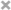### Markdown in Github

stack overview上的回答中的解释：

GitHub中markdown的解析是由SunDown库完成的，这个库的一个设计原则就是：

“Standards compliant, fast, secure markdown processing library in C”

### 在GFM中插入公式的方法

#### 1.最佳方案-Script+MathJax

MathJax是一个用于数学公式展示的JavaScript引擎，它支持几乎全部浏览器。

<script src="https://cdn.mathjax.org/mathjax/latest/MathJax.js?config=TeX-AMS-MML_HTMLorMML" type="text/javascript"></script>


MathJax默认行内公式标记为”\\“，可以使用以下方法来支持”$”符号(注意将下面的代码加到上面代码之前)： <script type="text/x-mathjax-config"> MathJax.Hub.Config({ tex2jax: { inlineMath: [ ['$','\$'], ["\$","\$"] ],
processEscapes: true
}
});
</script>


#### 2.使用外部网站来将公式渲染为图片

<img src="http://chart.googleapis.com/chart?cht=tx&chl= 在此插入Latex公式" style="border:none;">


<img src="http://chart.googleapis.com/chart?cht=tx&chl=\Large x=\frac{-b\pm\sqrt{b^2-4ac}}{2a}" style="border:none;">##### 使用forkosh服务器

<img src="http://www.forkosh.com/mathtex.cgi? 在此处插入Latex公式">


<img src="http://www.forkosh.com/mathtex.cgi? \Large x=\frac{-b\pm\sqrt{b^2-4ac}}{2a}">